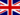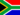# Triangoli angolari - Ottuso

• Note di Apprendimento
• Revisione degli argomentiLEENA P. 0 3 1 angle is known to be 90 degrees and the other 2 are less then the 1st angle.Isabel N. 0 2 one angle measures more than 90 degrees but two angles measures less than 90 degreesAnnette S. 0 2 Obtuse triangles have sides that may or may not be equal in length. The two acute angles must be equal in length.Dhurba R. 0 2 one angle that is measured more than 90 degrees and two angles are measured lessDonna M. 0 2 1 angle measures more than 90 degrees & 2 measure less
• Text Version